# Xcos vs. Simulink® – Logic and Bit Operations library conversion

In the table below you can find the equivalent between the Simulink® and Xcos blocks for Logic and Bit Operations library/palette. The blocks which are not present in both libraries are omitted from the table.

This conversion table can be used in case you need to convert a Simulink® model to an Xcos block diagram model or vice-versa.

 Simulink Logic and Bit Operations library Xcos Integer palette Xcos block description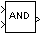Logical Operator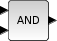*Logical operation This block is found in the palette “Commonly used blocks palette” and not Integer. The Logical Operator block performs the specified logical operation on its inputs. An input value is TRUE (1) if it is nonzero and FALSE (0) if it is zero.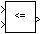Relational Operator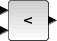*Relational operation This block is found in the palette “Commonly used blocks palette” and not Integer. It performs logical comparison of its two inputs.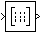Combinatorial Logic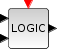Combinatorial Logic This block implements a standard truth table for modeling programming array, digital circuit and any other Boolean expressions. The user must specify a matrix that defines all the possible block outputs in the Truth Table field. In consequence, the number of rows must be a power of two. Each row of the matrix contains a logic combination of input elements.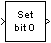Bit Set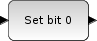Set a Bit This block sets to 1 the specified bit of its input. The user gives the bit index in the field Index of Bit with index 0, as that of the least significant bit.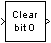Bit Clear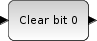Clear a Bit This block sets to 0 the specified bit of its input. The user gives the bit index in the field Index of Bit with index 0, as that of the least significant bit.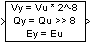Shift Arithmetic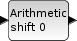Shift/Rotates Bits This block shifts the bits of the input signal. In this operation the digits are moved to the right or to the left. The user can choose the rule to shifts the bits that can be normal or cycle by setting the Shift Type parameter to 0 or 1. The number and the direction of the shifts are set with the Number of Bits to Shift Left. If this number is positive the input is shifted to the left, otherwise it is shifted to the right.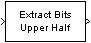Extract Bits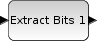Bits Extraction For an integer input this block outputs a contiguous selection of bits. It’s performing a bitwise AND between Input and Mask an integer of the same length as input with the bits to extract set to 1 and the other bits set to 0.

* These blocks are found in the Xcos palette “Commonly used blocks palette”

Let’s look at a simple example in which we create a Simulink® block diagram model, simulate it and display the results. We’ll recreate the equivalent model in Xcos and check if the results are the same.

### Simulink® block diagram model

The purpose of the model is only to use some of the Logic and Bit Operations Simulink® blocks which have an equivalent in the Integer Xcos palette.

The main parameters of each block are visible on the block’s icon. The same parameters are going to be used for the Xcos block diagram.

### Xcos block diagram model

We follow the same approach for the Xcos block diagram model. Using equivalent blocks (see table above), we recreate the same functionality of the Simulink model.

The block parameters have the same (equivalent) values as those in the Simulink block diagram.

As you can see, the output of the Xcos block diagram model are identical with the Simulink result. This proves that we can use Xcos as an alternative to Simulink, when we want to simulate and analyze logic and bit operations models.

For any questions, observations and queries regarding this article, use the comment form below.

Don’t forget to Like, Share and Subscribe!

#### Ad Blocker Detected

Dear user, Our website provides free and high quality content by displaying ads to our visitors. Please support us by disabling your Ad blocker for our site. Thank you!